# Odd Numbers

## Introduction

The word “odd” does not have its origin in mathematics. Oddly, the word comes to us from geography. Odd comes from the Old Norse word oddi, meaning "point of land." Points of land so designated in Old Norse being roughly triangular, oddi was over time generalized to mean "triangle." Since a point of land can be seen as the apex, or tip, of a triangle, with the other two angles forming a pair at the base, the word oddi was extended to mean "odd number"—the item of a threesome left over after the other two items are paired off.

## Odd numbers

Odd numbers are whole numbers that cannot be exactly divided by $$2.$$

Let’s take $$7$$ red-colored counters.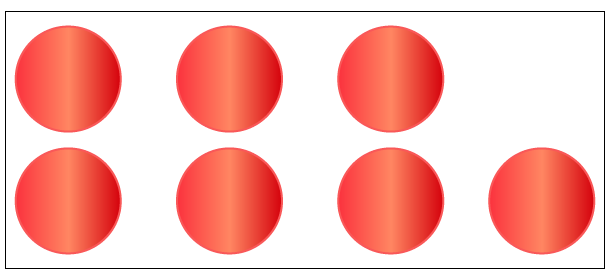Let’s try and pair the counters.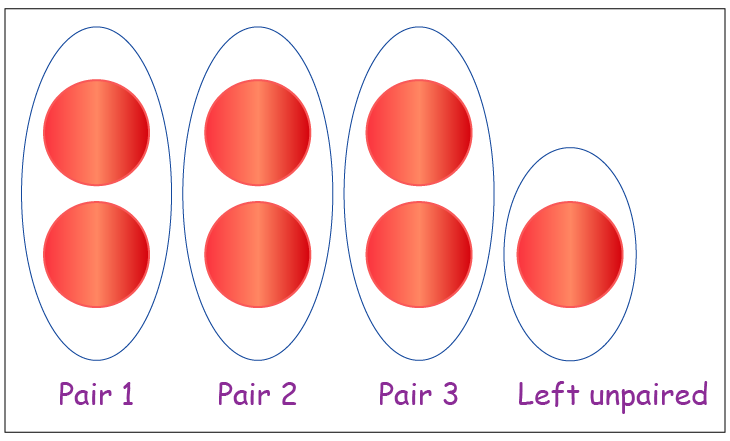We are able to pair $$6$$ counters and form $$3$$ pairs.
The $$7^\text{th}$$ counter cannot be paired. It is left unpaired.
Thus, we can conclude that $$7$$ is an odd number.

Let’s divide $$7$$ by $$2.$$
We get the quotient as $$3,$$ which is equal to the number of pairs that are formed.
We get the remainder as $$1,$$ which is equal to the number of counters that cannot be paired.

Whenever we try to pair an odd number of counters, we will always be left with $$1$$ counter that cannot be paired.
Whenever an odd number is divided by $$2,$$ we will always get the remainder as $$1.$$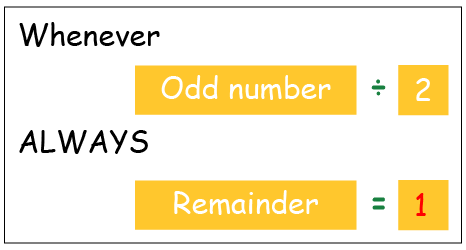Note: An odd number always ends in $$1, 3, 5, 7$$ or $$9.$$

## Odd and even numbers

Let’s perform addition on different combinations of odd and even numbers.

 Number $$1$$ Number $$2$$ Sum $$2$$ [Even] $$8$$ [Even] $$10$$ [Even] $$1$$ [Odd] $$3$$ [Odd] $$4$$ [Even] $$5$$ [Odd] $$6$$ [Even] $$11$$ [Odd] $$8$$ [Even] $$9$$ [Odd] $$17$$ [Odd]

Let’s summarize the result.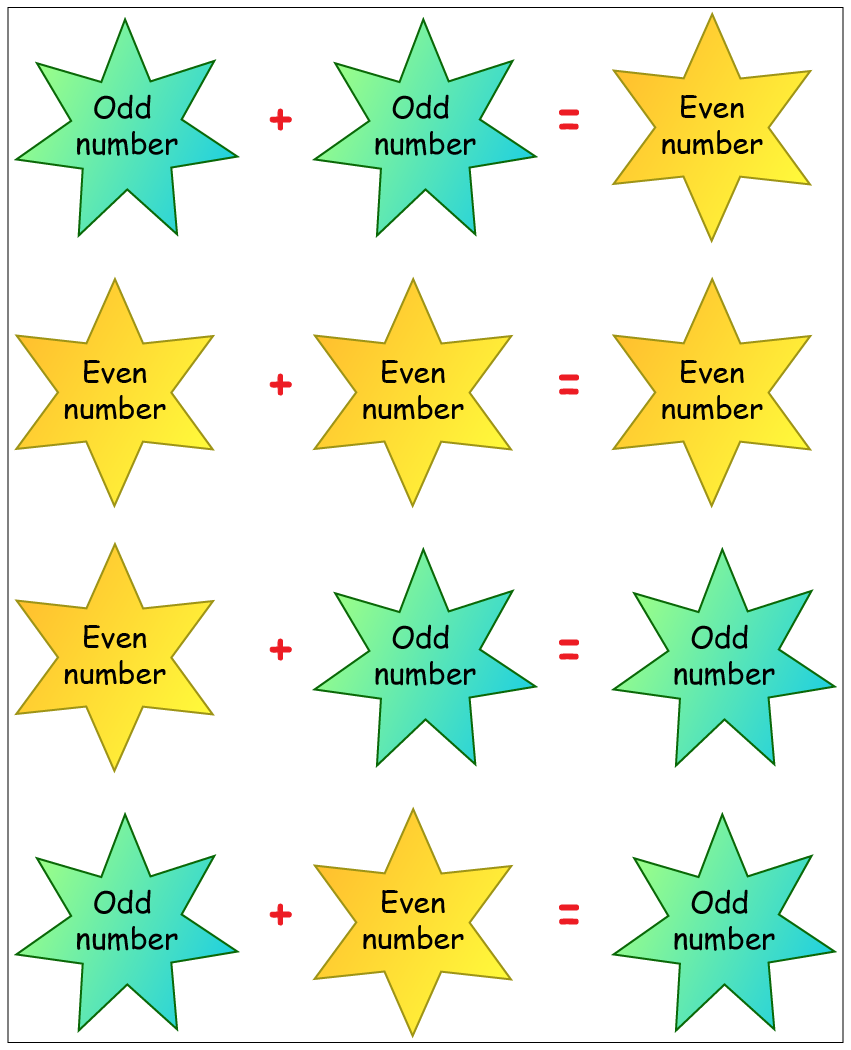You can also learn the properties of ODD and EVEN numbers, the CUEMATH way with the help of this simple video!

## Solved Examples

### Example 1:

Find whether the number of objects given in the box is odd or even.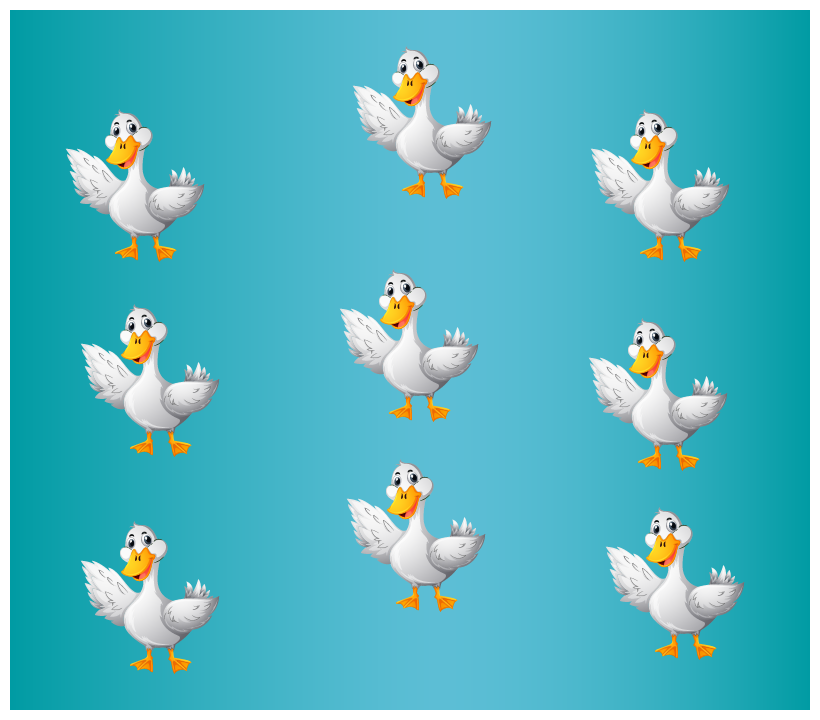Step 1:

Count the number of objects.
Number of ducks $$= 9$$

Step 2:

Pair the objects.
As shown below, there are $$4$$ pairs of ducks.
$$1$$ duck cannot be paired.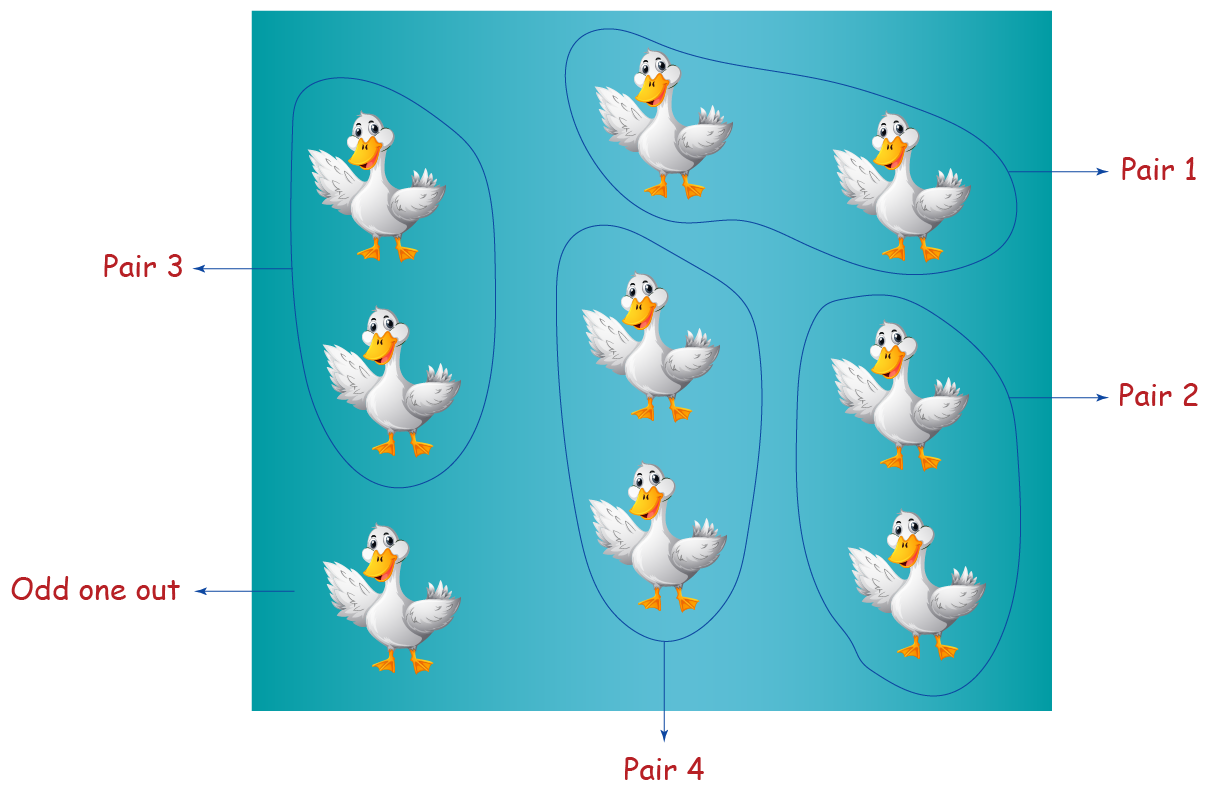Step 3:

If all the objects cannot be paired then the number of objects represents an ODD number.

Thus, $$9$$ is an ODD number.

## Practice Questions

1)  Circle the odd numbers
(a)  $$713\qquad358\qquad620\qquad797\qquad249$$
(b)  $$2233\quad3461\quad8271\quad4210\quad7181$$
(c)  $$8944\quad8727\quad9546\quad8391\quad9569$$
(d)  $$4810\quad6069\quad8670\quad9719\quad9900$$

Hint: An odd number always ends in $$1, 3, 5, 7$$ or $$9.$$

2)  Which two digits of the number $$6852$$ must be interchanged to make it an odd number?
Hint: An odd number always ends in $$1, 3, 5, 7$$ or $$9.$$

3)  Which of these numbers when added to an even number results in an odd number?
(a)  $$21$$         (b) $$42$$         (c) $$68$$         (d) $$102$$

Hint: Even + Odd = Odd.

More Important Topics
Numbers
Algebra
Geometry
Measurement
Money
Data
Trigonometry
Calculus
More Important Topics
Numbers
Algebra
Geometry
Measurement
Money
Data
Trigonometry
Calculus
Learn from the best math teachers and top your exams

• Live one on one classroom and doubt clearing
• Practice worksheets in and after class for conceptual clarity
• Personalized curriculum to keep up with school